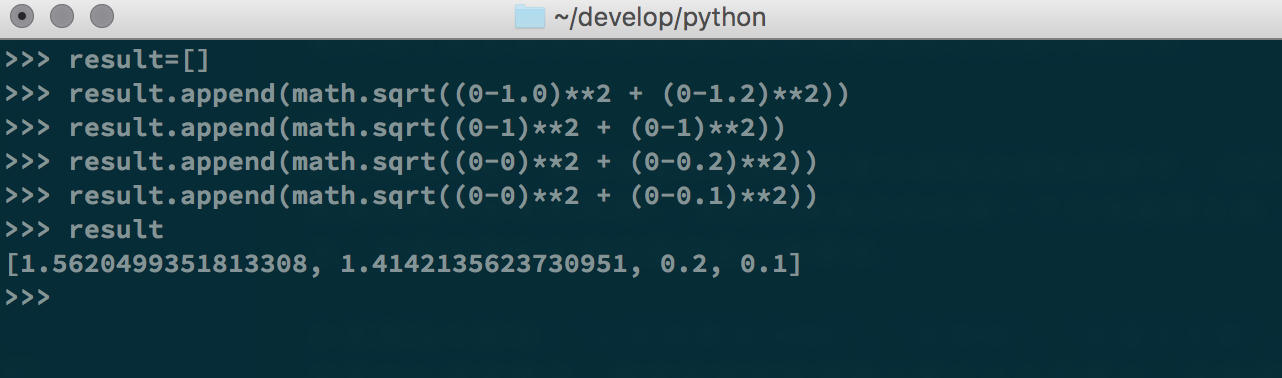## 分类算法

### k-近邻算法$d = \sqrt{(x_{1} - x_{2})^2 + (y_{1} - y_{2}) ^2 + (z_{1} - z_{2})^2 + … + (i_{1} -i_{2})^2}$

### 条件概率与贝叶斯

DeepMind 星际争霸 学术

Apple 交互 设计

P(学术|谷歌x星际争霸)
= P(谷歌|学术) x P(星际争霸|学术) x P(学术)/(P(谷歌) x P(星际争霸))

A 1 0 1 0
B 0 1 0 1
C 1 0 1 1